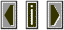Morphologya morphology is an "ordered set" of values.  Larry Polansky adopted the term morphology from James Tenney's Meta + Hodos.

 Morphological features differ from statistical features in that they contain a profile. The profile of a morphology is derived from the order of the components of the set. Statistical features, in contrast, generally refer to the global properties such as mean and range values of a parameter.

 Each of the integers in an ordered set bears some relationship to that which precedes it and that which follows it. The theory of morphological metrics, or the study of formal distances, is something Polansky applies to the mutation functions and is an important paradigm to understand if one wishes to understand how mutation works. Polansky identifies Hauer and Schoenberg as the first twentieth century composers to work with ideas of 'ordered sets' in music (Hauer's tropes and Schoenberg's 12-tone rows). Polansky, in his paper "Morphological Metrics: An Introduction to the Theory of Formal Distances" outlines some of the basics of morphological metrics. Some of these metrics precede the mutation functions and may help to understand how mutation works by using a theory of formal distances.

 ordered metric: unordered metric: linear metric: one in which the sequence of values is retained in the calculation of distance. ones which do not use the sequence of values. a metric is linear if in the calculation of distance, the number of intervals used within either of the morphologies in the calculation of a metric is less than or equal to L-1 (where L is the length of the metric). combinatorial: directional: magnitudinal: a metric is combinatorial if within one of the two morphologies, more than L-1 intervals are computed. Combinatoriality is complex and there is a wide range of cominatorial possibilities for all metrics of two morphologies. a metric is directional if it considers the sign of an interval in a morphology. a metric is magnitudinal if it considers the value of the interval. Magnitudinal metrics may be further categorized into intervallic or absolute. In general, absolute metrics do not make interval calculations within a morphology.

 In general, morphological metrics are designed as a manner of calculating distance and similarity between two morphologies. As Tenney and Polansky would state, these concepts are fundamental to the discussion of form.

 Morphological mutations and their applications in SoundHack are based upon these theories of distances with the differences between frequency bands in successive FFT analysis frames acting as the elements of a morphology. Using the FFT analysis of two sounds, intervalic distances may be created and similarities compared. In this way, elements of a source morphology may be blended in some fashion with elements of a target morphology to result in a mutant.